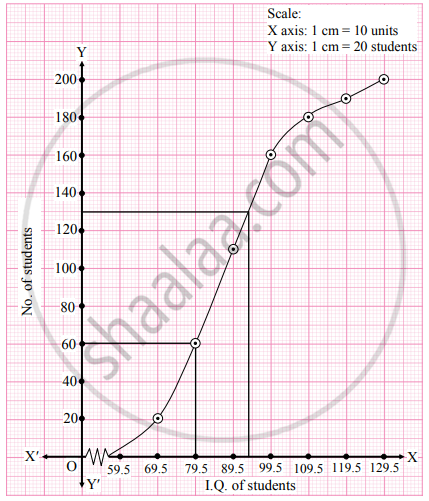# Find graphically the values of D3 and P65 for the data given below: I.Q of students 60 – 69 70 – 79 80 – 89 90 – 99 100 –109 110 –119 120 –129 No. of students 20 40 50 50 20 10 10 - Mathematics and Statistics

Graph

Find graphically the values of D3 and P65 for the data given below:

 I.Q of students 60 – 69 70 – 79 80 – 89 90 – 99 100 – 109 110 – 119 120 – 129 No. of students 20 40 50 50 20 10 10

#### Solution

Since the given data is not continuous, we have to convert it in the continuous form by subtracting 0.5 from the lower limit and adding 0.5 to the upper limit of every class interval. To draw a ogive curve, we construct the less than cumulative frequency table as given below:

 I.Q. of students No. of students(f) Less than cumulative frequency(c.f.) 59.5 – 69.5 20 20 69.5 – 79.5 40 60 79.5 – 89.5 50 110 89.5 – 99.5 50 160 99.5 – 109.5 20 180 109.5 – 119.5 10 190 119.5 – 129.5 10 200 Total 200

Points to be plotted are (69.5, 20), (79.5, 60), (89.5, 110), (99.5, 160), (109.5, 180), (119.5, 190), (129.5, 200).N = 200

For D3, (3"N")/10=(3xx200)/10 = 60

For P65, (65"N")/100=(65xx200)/100 = 130

∴ We take the values 60 and 130 on the Y-axis. From these points we draw lines parallel to X-axis and from the points where these lines intersect less than ogive, we draw perpendiculars on X-axis. The foot of perpendiculars represents the median of the values, D3, and P65.

∴ D3 = 79.5, P65 = 93.5

Concept: Graphical Location of Partition Values
Is there an error in this question or solution?
Chapter 1: Partition Values - Exercise 1.3 [Page 20]

Share